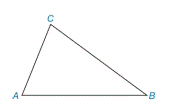Chapter 2.4, Problem 3EElementary Geometry For College St...

7th Edition
Alexander + 2 others
ISBN: 9781337614085

Solutions

Chapter
SectionElementary Geometry For College St...

7th Edition
Alexander + 2 others
ISBN: 9781337614085
Textbook Problem

In Exercise 1 to 4, refer to Δ A B C . On the basis of the information given, determine the measure of the remaining angle(s) of the triangle.m ∠ A = m ∠ C = 67 °

To determine

To find:

The measure of B.

Explanation

Given:

The given statement is,

mA=mC=67°

Figure (1)

Calculation:

In a triangle, the sum of the measures of the interior angles is 180°.

The given statement is,

mA=mC=67°

The sum of the measures of the interior angles is 180°.

mA+mB+mC=180°

Substit

Still sussing out bartleby?

Check out a sample textbook solution.

See a sample solution

The Solution to Your Study Problems

Bartleby provides explanations to thousands of textbook problems written by our experts, many with advanced degrees!

Get Started

In Exercise 11-14, factor the expression. y27y+494

Calculus: An Applied Approach (MindTap Course List)

Convert the expressions in Exercises 6584 to power form. x2x

Finite Mathematics and Applied Calculus (MindTap Course List)

What is wrong with the equation? /3sectand=sec]/3=3

Single Variable Calculus: Early Transcendentals

Using a binomial series, the Maclaurin series for is:

Study Guide for Stewart's Single Variable Calculus: Early Transcendentals, 8th